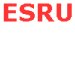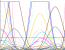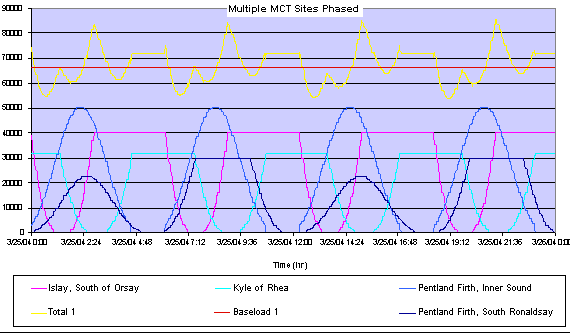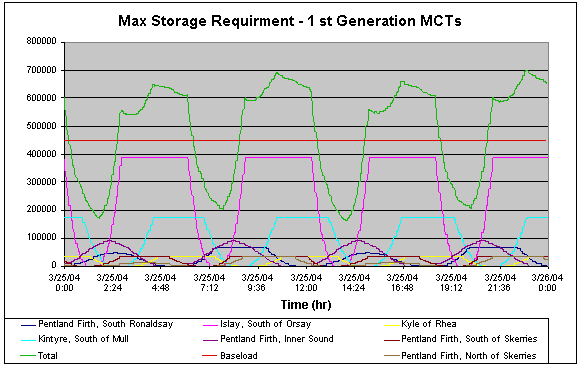Phasing multiple MCT sitesMethodology

Once the sites were selected, the number of MCT units to be installed at each site was chosen and the resulting power output was phased together on one graph, as can be seen in the figures below. The combined total power output was then calculated.Figure: The power output from multiple sites phased together

Selecting the number of MCT units determined the installed capacity of each site and in turn specified the total power output. The amount of pumped storage and pumped generation was then selected  as detailed in the Use of Storage section - to determine the storage requirement and the size of the baseload that could be provided from the sites selected.

To ensure the least variation in the total output, the installed capacity of each site was limited by the capacity of the smallest sites, in this case the Kyle of Rhea and the Pentland Firth, Inner Sound. This was the principle behind the initial Baseload Supply Strategy scenario, which aimed to minimise the required pumped storage through reducing the variation in total output, as can be seen in the figure above.

To maximise the potential baseload that could be supplied, greater capacities were selected at the larger sites, such as Islay and Kintyre. However, this would increase the variation in the total output - the figure below shows an increased variation of some 50MW - and hence increase the amount of storage required. This was the basis for the second Supply Strategy scenario, which aimed to maximise the potential power output and generate the largest baseload possible, as shown in the figure below.Figure: Baseload Supply Strategy, maximum storage requirement scenario with large variation in total output